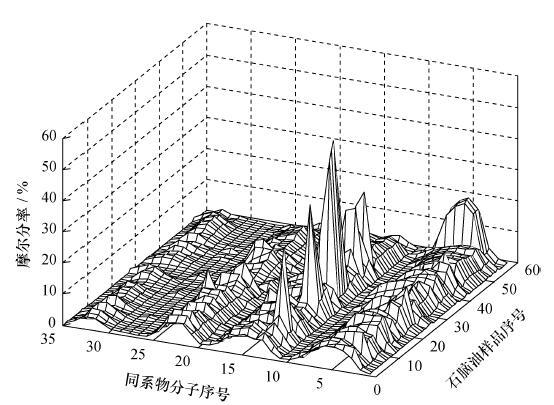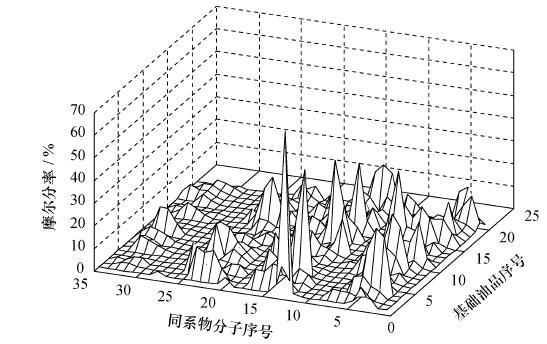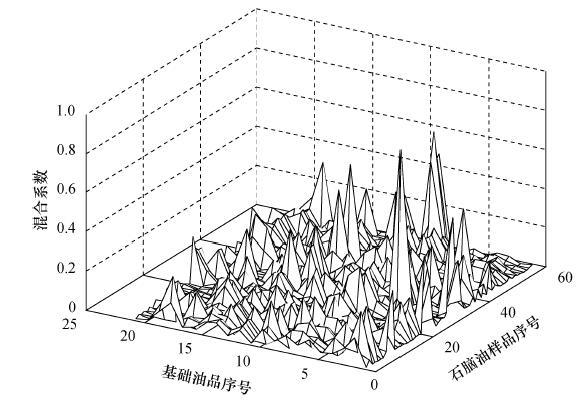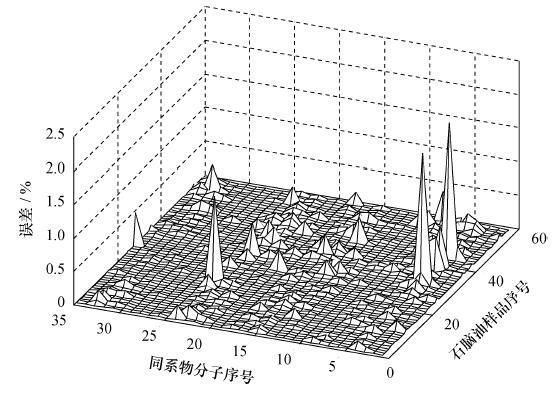, 杜玉鹏 , 王振雷 , 钱锋

Naphtha characterization based on a molecular-type homologous series vector representation
, DU Yupeng , WANG Zhenlei , QIAN Feng
Key Laboratory of Advanced Control and Optimization for Chemical Processes of the Ministry of Education, East China University of Science and Technology, Shanghai 200237, China
Abstract:A novel homologous series vector representation method was developed for naphtha in which each homologous molecule of naphtha is defined as a state variable and all these variables are then used to construct a high dimension vector space. Thus, any variation of naphtha as one point in this vector space can be blended linearly by a group of independent naphthas named Basis Oils. These basis oils are obtained using the non-negative matrix factorization (NMF) method with the components data matrix of a huge number of naphtha samples factorized into a characteristic matrix with a lower dimension and its coefficient matrix. In a case study, a naphtha model containing 21 groups of naphtha bases was extracted from 59 groups of naphtha samples with a maximum representation error of less than 2.5 percent of the original data.
Key words: naphtha     detailed group components     molecular-type homologous series vector representation     non-negative matrix factorization (NMF)

MTHS矩阵表征法的特点在于将石油烃内的分子组成分布情况在一个三维空间内直观地呈现出来。然而石脑油包含有几十种分子同系物，同系物分布的不同将使得石脑油表现出不同的特性，这种差异性在三维空间内很难进行定量刻画。因此，本文提出一种石脑油向量表征方法，在此基础上运用非负矩阵分解方法提取石脑油分子组成分布的共性特征。

1 石脑油馏分的向量表征

 \begin{align} & \text{NA}{{\text{P}}_{\text{k}}}=\sum\limits_{i=3}^{12}{\left( a_{i}^{k}\cdot \text{n}{{\text{P}}_{i}}+b_{i}^{k}\cdot \text{i}{{\text{P}}_{i}}+c_{i}^{k}\cdot {{O}_{i}}+ \right.} \\ & \left. d_{i}^{k}\cdot \text{N}{{\text{a}}_{i}}+e_{i}^{k}\cdot {{A}_{i}} \right). \\ \end{align} (1)

 \begin{align} & \text{NA}{{\text{P}}_{\text{k}}}=\left( \begin{matrix} \text{n}{{\text{P}}_{i}} & \text{i}{{\text{P}}_{i}} & {{O}_{i}} & \text{N}{{\text{a}}_{i}} & {{A}_{i}} \\ \end{matrix} \right). \\ & {{\left( a_{i}^{k},b_{i}^{k},c_{i}^{k},d_{i}^{k},e_{i}^{k} \right)}^{\text{T}}} \\ & \triangleq S\cdot {{w}_{k}}. \\ \end{align} (2)

 $M={{\begin{matrix} a_{i}^{1} & a_{i}^{2} & \cdots & a_{i}^{m} \\ b_{i}^{1} & b_{i}^{2} & {} & b_{i}^{m} \\ c_{i}^{1} & c_{i}^{2} & {} & c_{i}^{m} \\ d_{i}^{1} & d_{i}^{2} & {} & d_{i}^{m} \\ e_{i}^{1} & e_{i}^{2} & \cdots & e_{i}^{m} \\ \end{matrix}}_{n\times m}}.$ (3)

m=n，则子空间M即是原料状态空间S，这就意味着任意一种石脑油都是这m种石脑油的一个线性组合。换而言之，可由这m种石脑油按照某种特定比例混合得到任意一种石脑油，因此，这m种石脑油也被称为基础油品。由于石脑油中组分种类繁多，要找到整个原料状态空间内的基础油品几乎不可能，但在实际应用中所涉及的石脑油样品的馏程范围相对比较固定。因此，只需要用一个维数较小的原料子空间内来刻画实际应用中所涵盖的石脑油样品特性。

 $\text{NAP=}\sum\limits_{k=1}^{m}{{{h}_{k}}\cdot \text{NA}{{\text{P}}_{k}}=\sum\limits_{k=1}^{m}{S\cdot \left( {{h}_{k}}{{w}_{k}} \right)=S\cdot v,}}$ (4)
 \begin{align} & v=\sum\limits_{k=1}^{m}{{{h}_{k}}{{w}_{k}}=\left[ {{w}_{1}},{{w}_{2}},\cdots ,{{w}_{m}} \right]\cdot {{\left[ {{h}_{1}},{{h}_{2}},\cdots ,{{h}_{m}} \right]}^{\text{T}}}}= \\ & W\cdot {{h}^{\text{T}}}. \\ \end{align} (5)

2 基于非负矩阵分解NMF的石脑油特征提取

 $V={{\begin{matrix} a_{i}^{1} & a_{i}^{2} & \cdots & a_{i}^{N} \\ b_{i}^{1} & b_{i}^{2} & {} & b_{i}^{N} \\ c_{i}^{1} & c_{i}^{2} & {} & c_{i}^{N} \\ d_{i}^{1} & d_{i}^{2} & {} & d_{i}^{N} \\ e_{i}^{1} & e_{i}^{2} & \cdots & e_{i}^{N} \\ \end{matrix}}_{n\times N}}\triangleq \left[ \begin{matrix} {{v}_{1}} & {{v}_{2}} & \cdots & {{v}_{N}} \\ \end{matrix} \right].$ (6)

 \begin{align} & V=\left[ \begin{matrix} W\cdot h_{1}^{\text{T}} & W\cdot h_{2}^{\text{T}} & \cdots & W\cdot h_{\text{N}}^{\text{T}} \\ \end{matrix} \right]= \\ & {{W}_{n\times m}}\cdot {{H}_{m\times N}}. \\ \end{align} (7)

NMF分解最早由Lee和Seung提出，在图像分析、 文本聚类、 数据挖掘、 语音处理等方面得到了广泛应用。NMF可以用式(8)所示优化问题描述，即

 \begin{align} & \underset{W,H}{\mathop{\text{min}}}\,{{\left\| V-W\cdot H \right\|}_{F}}, \\ & \text{s}\text{.t}\text{.}W\in \mathbb{R}_{+}^{n\times m},H\in \mathbb{R}_{+}^{m\times N}. \\ \end{align} (8)

Step 1 基础油品维数的确定。

Step 2WH的初始化。

Step 3 根据乘性迭代准则计算WH

 \begin{align} & H\leftarrow H\cdot \left( {{W}^{\text{T}}}\left( V/\left( W\cdot H \right) \right) \right), \\ & W\leftarrow W\cdot \left( \left( V/\left( W\cdot H \right)\cdot {{H}^{\text{T}}} \right) \right), \\ & {{W}_{m\times n}}\leftarrow \frac{{{W}_{m\times n}}}{{{\sum }_{m}}{{W}_{m\times n}}}. \\ \end{align}

Step 4 误差判断。

3 实例研究图 1 石脑油样品组成分布情况图 2 基础油品组成分布情况图 3 石脑油样品关于基础油品的混合系数分布情况图 4 重构油品与样品组成误差分布

4 结论

  Vendeuvre C, Bertoncini F, Duval J L, et al. Comparison of conventional gas chromatography and comprehensive two-dimensional gas chromatography for the detailed analysis of petrochemical samples[J]. Journal of Chromatography A , 2004, 1056 (1-2) : 331–347.  Qian K N, Dechert G J. Recent advances in petroleum characterization by GC field ionization time-of-flight high-resolution mass spectrometry[J]. Analytical Chemistry , 2002, 74 (16) : 3977–3983. DOI:10.1021/ac020166d  Pyl S P, Van Geem K M, Reyniers M, et al. Molecular reconstruction of complex hydrocarbon mixtures:An application of principal component analysis[J]. AIChE Journal , 2010, 56 (12) : 3174–3188. DOI:10.1002/aic.v56:12  Saine Aye M, Zhang N. A novel methodology in transforming bulk properties of refining streams into molecular information[J]. Chemical Engineering Science , 2005, 60 (23) : 6702–6717. DOI:10.1016/j.ces.2005.05.033  Van Geem K M, Hudebine D, Reyniers M F, et al. Molecular reconstruction of naphtha steam cracking feedstocks based on commercial indices[J]. Computers and Chemical Engineering , 2007, 31 (9) : 1020–1034. DOI:10.1016/j.compchemeng.2006.09.001  Dente M, Ranzi E. Detailed prediction of olefin yields from hydrocarbon pyrolysis through a fundamental simulation program (SPYRO)[J]. Computers and Chemical Engineering , 1979, 3 (1-4) : 61–75. DOI:10.1016/0098-1354(79)80013-7  Speight J G. The Chemistry and Technology of Petroleum,[M]. New York, USA: Marcel Dekker, 1998 .  Riazi M R. Characterisation and Properties of Petroleum Fractions[M]. Philadelphia, USA: ASTM International, 2005 .  PENG Bin. Molecular Modeling of Refinery Process[D]. Manchester, UK:The University of Manchester, 1999.  ZHANG Yan. A Molecular Approach for Characterization and Property Predictions of Petroleum Mixtures with Applications to Refinery Modeling[D]. Manchester, UK:The University of Manchester, 1999.  Ahmad M I, Zhang N, Jobson M. Molecular components-based representation of petroleum fractions[J]. Chemical Engineering Research and Design , 2011, 89 : 410–420. DOI:10.1016/j.cherd.2010.07.016  Lee D, Seung H. Learning the parts of objects by non-negative matrix factorization[J]. Nature , 1999, 401 : 788–791. DOI:10.1038/44565